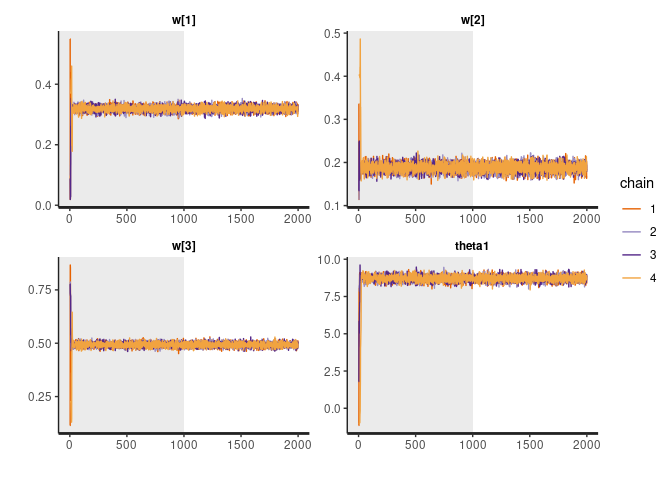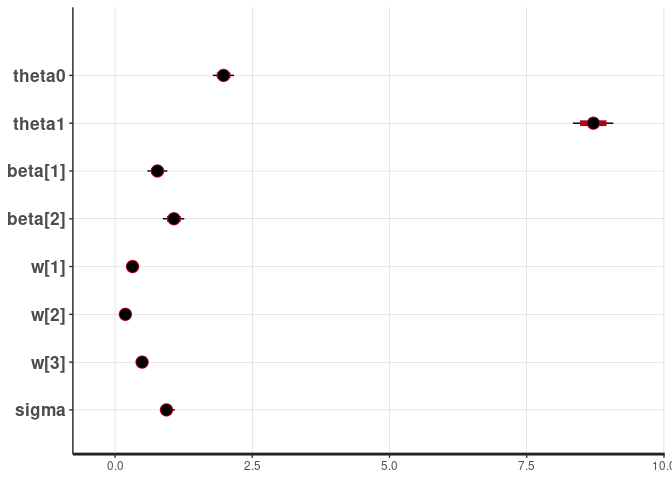# bws

The goal of bws is to provide a user-friendly and efficient implementation of the Bayesian Weighted Sums (BWS) described by Hamra, Maclehose, Croen, Kauffman, and Newschaffer (2021) with some extensions to work with binary and count response data.

## Installation

You can install the development version from GitHub with:

``````# install.packages("devtools")
devtools::install_github("phuchonguyen/bws")``````

## Example

This is a basic example which shows you how to fit BWS:

``````## We first need to simulate some data
set.seed(123)
N <- 100
P <- 3
K <- 2
X <- matrix(rnorm(N*P), N, P)
Z <- matrix(rnorm(N*K), N, K)  # confounders
w <- c(0.3, 0.2, 0.5)
theta0 <- 2
theta1 <- 3
beta <- runif(K, 0.5, 1.5)
y <- theta0 + theta1*theta1*(X%*%w) + Z%*%beta + rnorm(N)
## Fitting BWS is simple
fit <- bws::bws(iter = 2000, y = y, X = X, Z = Z,
chains = 4, cores = 2, show_messages = FALSE)
#> Warning: replacing previous import 'lifecycle::last_warnings' by
#> Warning: replacing previous import 'lifecycle::last_warnings' by

Since the implementation uses Stan and returns an `rstanfit` object, users can enjoy all the functionalities provided in `rstan` to analyze the fitted model:

``rstan::traceplot(fit, pars = c("w", "theta1"), inc_warmup = TRUE, nrow = 2)````````print(fit, pars = c("w", "theta1"))
#> Inference for Stan model: bws.
#> 4 chains, each with iter=2000; warmup=1000; thin=1;
#> post-warmup draws per chain=1000, total post-warmup draws=4000.
#>
#>        mean se_mean   sd 2.5%  25%  50%  75% 97.5% n_eff Rhat
#> w   0.32       0 0.01 0.30 0.31 0.32 0.33  0.34  5292    1
#> w   0.19       0 0.01 0.17 0.18 0.19 0.19  0.21  5750    1
#> w   0.49       0 0.01 0.47 0.49 0.49 0.50  0.51  6125    1
#> theta1 8.71       0 0.19 8.34 8.59 8.71 8.84  9.08  5388    1
#>
#> Samples were drawn using NUTS(diag_e) at Mon Jun 13 00:06:38 2022.
#> For each parameter, n_eff is a crude measure of effective sample size,
#> and Rhat is the potential scale reduction factor on split chains (at
#> convergence, Rhat=1).
rstan::plot(fit)
#> ci_level: 0.8 (80% intervals)
#> outer_level: 0.95 (95% intervals)``````The model inferred the correct weights, which are set to 0.3, 0.2, 0.5 in the simulation.

## Reference:

Hamra, G.B.; Maclehose, R.F.; Croen, L.; Kauffman, E.M.; Newschaffer, C. Bayesian Weighted Sums: A Flexible Approach to Estimate Summed Mixture Effects. International Journal of Environmental Research and Public Health 2021, 18, 1373. link